Question

The one-to-one function f is defined below. 8x-9 7x+4 Find f '(x), where s' is the...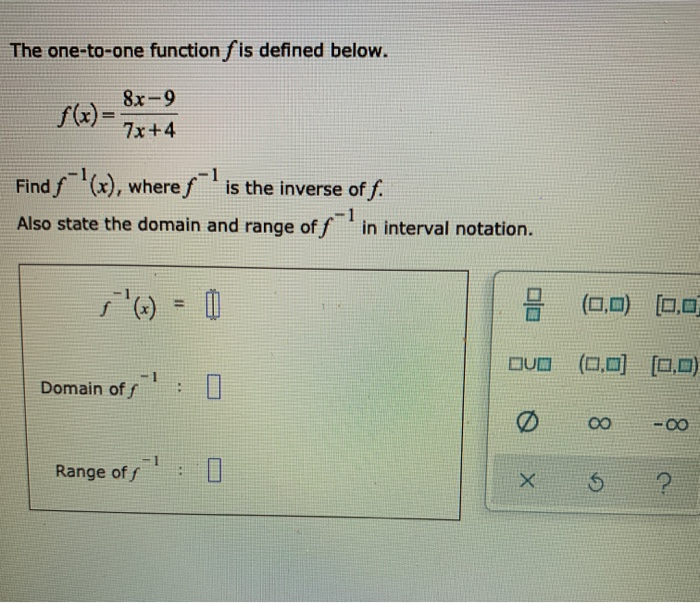The one-to-one function f is defined below. 8x-9 7x+4 Find f '(x), where s' is the inverse of f. Also state the domain and range of fin interval notation. (0,0) 0,0 DUD (0,0] [0,0) 1 - Domain off o -00 Range of X 5 ?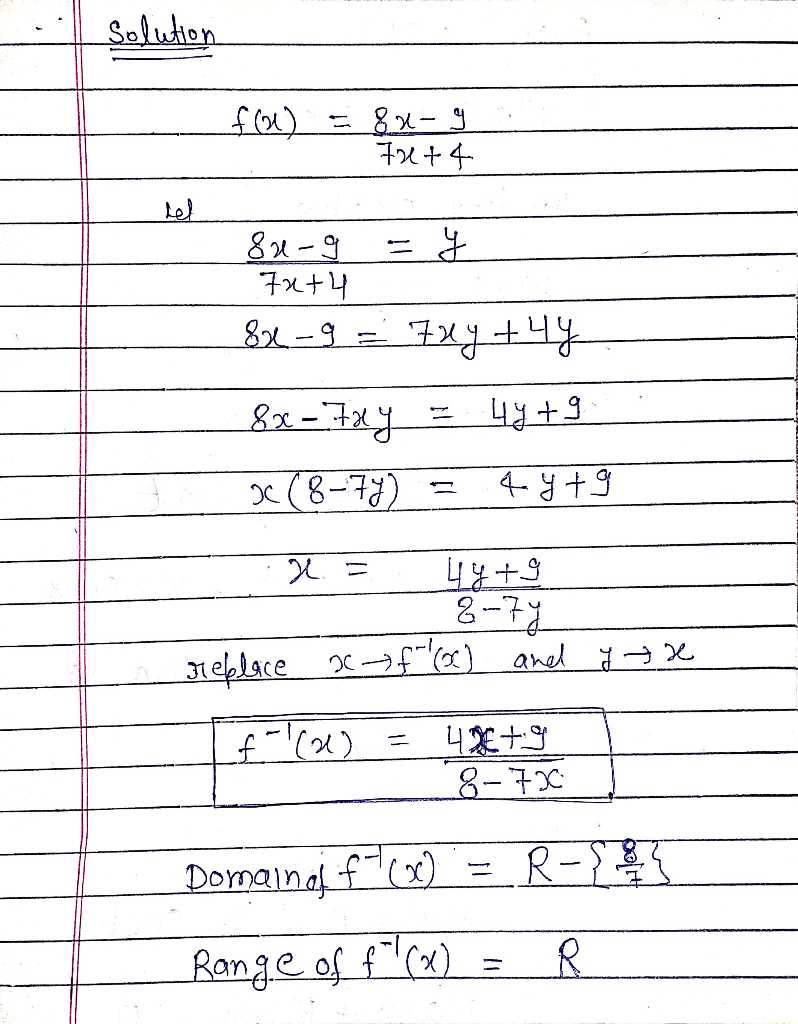Earn Coins

Coins can be redeemed for fabulous gifts.

Similar Homework Help Questions
• edge Check QL The one-to-one function f is defined below. 8x 7x-1 Find f '(x), where...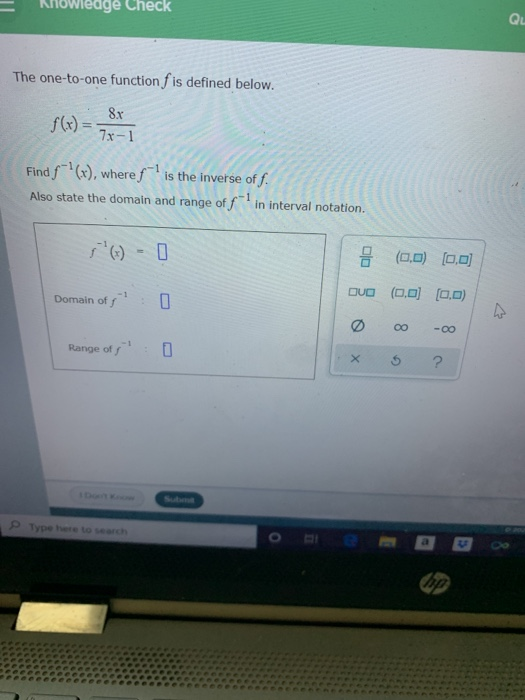edge Check QL The one-to-one function f is defined below. 8x 7x-1 Find f '(x), where fis the inverse of f. Also state the domain and range of fin interval notation. 0 (0,0) (0,0) - 1 Domain off Ovo (0,0) (0,0) 0 oo -00 Range of s 0 X ? Type here to search op

• Español The one-to-one function f is defined below. Find f '(x), where f-'is the inverse of...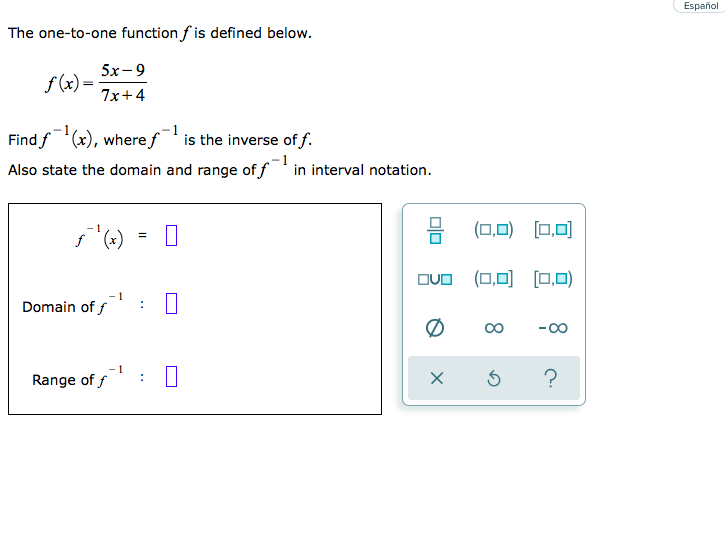Español The one-to-one function f is defined below. Find f '(x), where f-'is the inverse of f. Also state the domain and range of f" in interval notation. '(x) = 0 • (0,0) (0,0) duo (0,0) [0,0) Domain off' : 0 Range of 5' : 0 x 6 ?

• The one-to-one function f is defined below. 1. f(x) = 2 2 B Find f'(x), where...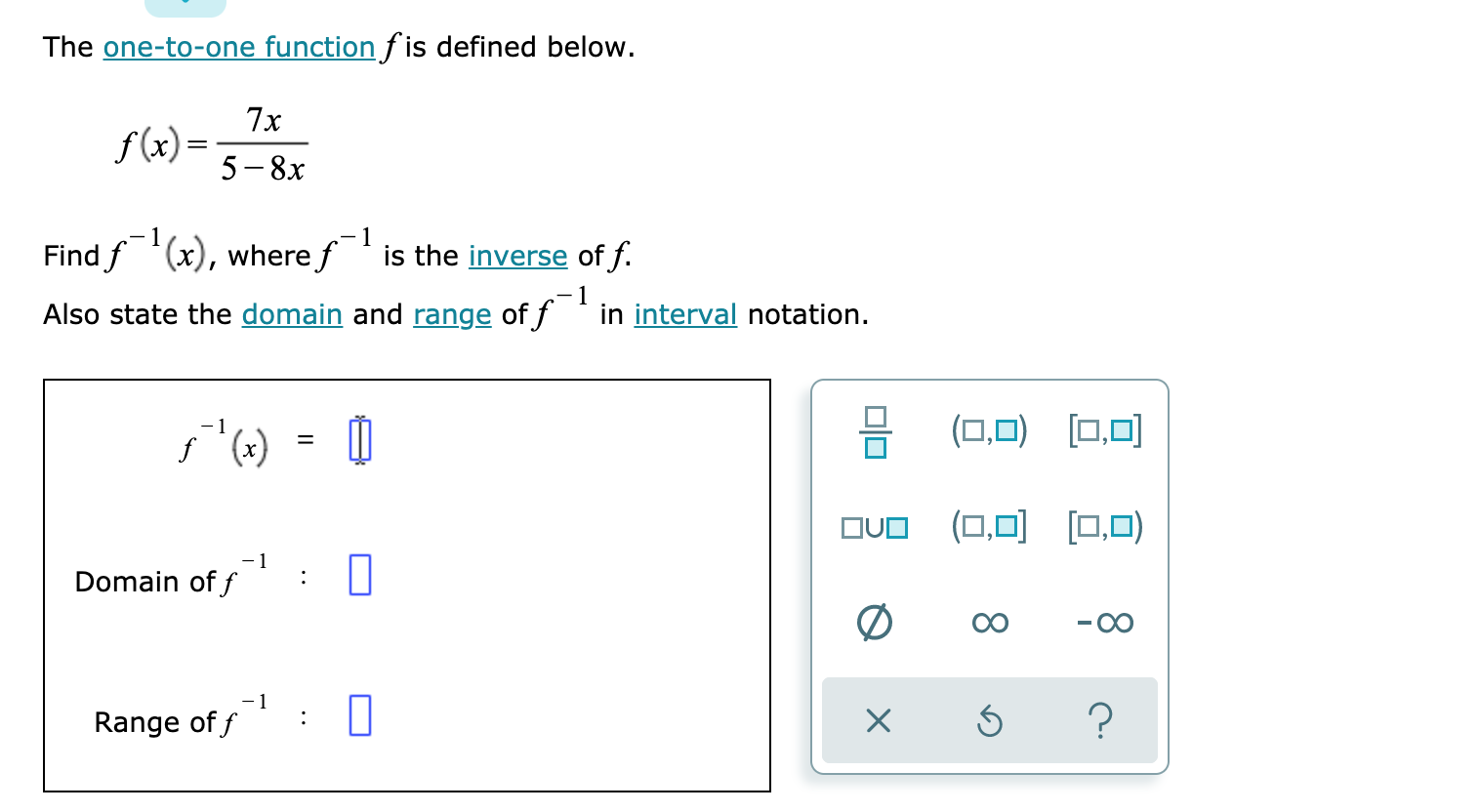The one-to-one function f is defined below. 1. f(x) = 2 2 B Find f'(x), where f is the inverse of f. Also state the domain and range of f in interval notation. 5 "(t) = 0 H (0,0) [0,0] OVO (0,0] [0,0) Domain of f : Ø -00 Range of f1? : 0 x 3 ?

• The one-to-one function f is defined below. \$(t)=5+Vx+10 Find f , the inverse off. Then give...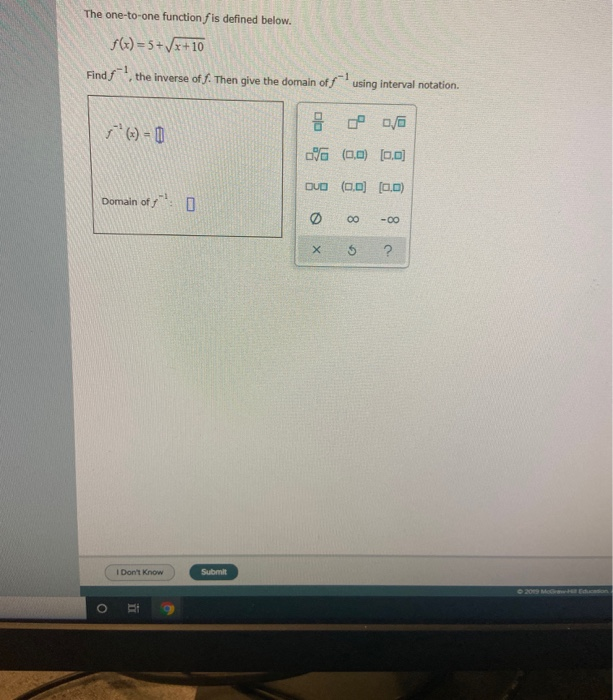The one-to-one function f is defined below. \$(t)=5+Vx+10 Find f , the inverse off. Then give the domain off using interval notation. lo (-) = 0 0/ VG (0,0) 0.0 oue (0.0 (0:0) 0 00 -00 Domain off o 68 * I Don't Know) Submit ori 9

• Consider the function /(x) = 5-x+8 for the domain (-,5). Find,'(), where is the inverse of...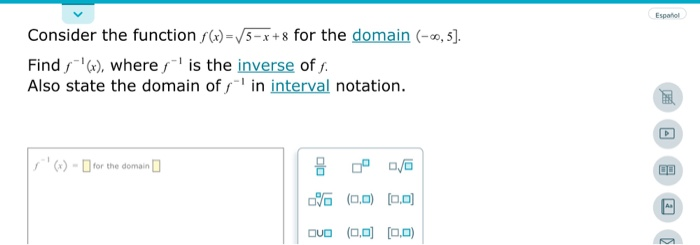Consider the function /(x) = 5-x+8 for the domain (-,5). Find,'(), where is the inverse of f. Also state the domain off' in interval notation. '() - for the domain no (0,0) (0,0) QUO (0,0) (0,0)

• = The one-to-one function f is defined below. f(x) = (x-9) Find f-'(x), where s-l is...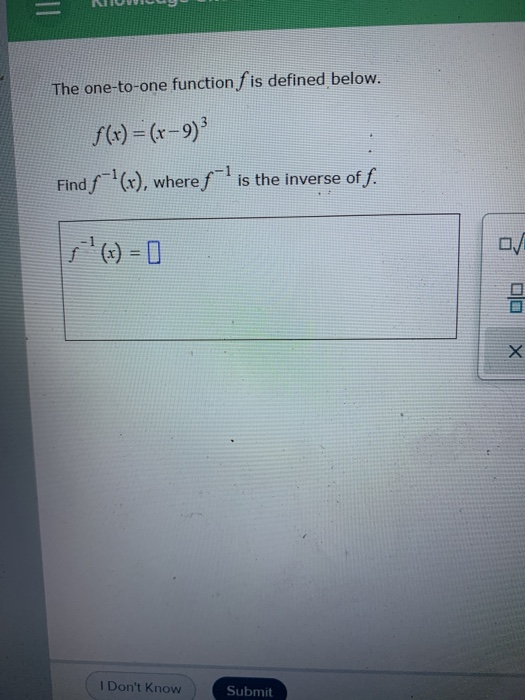= The one-to-one function f is defined below. f(x) = (x-9) Find f-'(x), where s-l is the inverse of f. DO X I Don't Know Submit

• The function f(x) = 3-7x is one-to-one. 5+11x (a) Find the inverse of f. (b) State...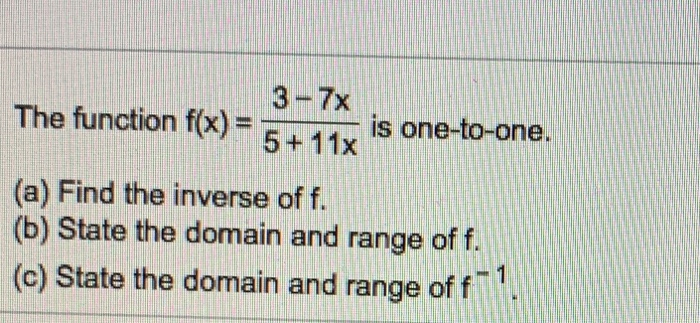The function f(x) = 3-7x is one-to-one. 5+11x (a) Find the inverse of f. (b) State the domain and range of f. (c) State the domain and range of f-1.

• Find the domain and the range of the function. 4 f(x)=1-7x+ 5, for x < 7....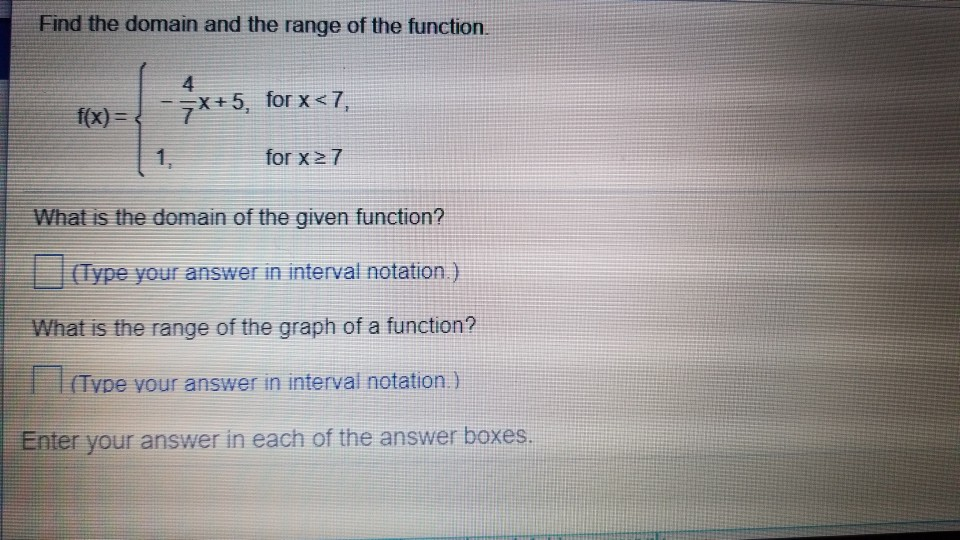Find the domain and the range of the function. 4 f(x)=1-7x+ 5, for x < 7. for x 27 What is the domain of the given function? (Type your answer in interval notation.) What is the range of the graph of a function? (Type your answer in interval notation.) Enter your answer in each of the answer boxes

• 25 (4 comple The functions f and g are defined as f(x) = 8x - 5...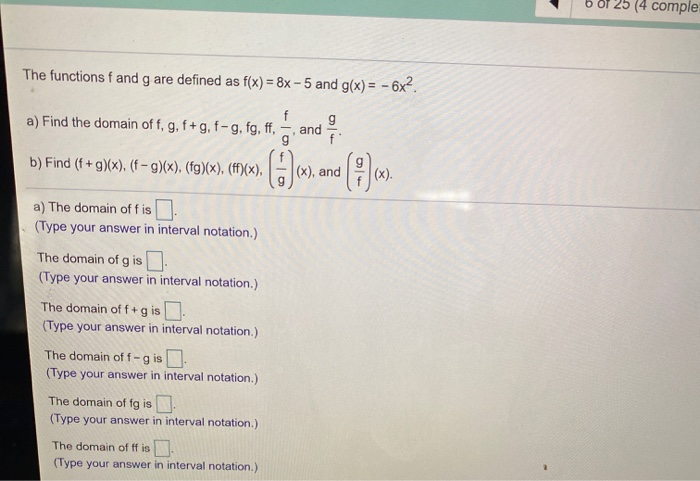25 (4 comple The functions f and g are defined as f(x) = 8x - 5 and g(x) = - 6x?. a) Find the domain of f, g, f+g, f-g, fg, ff, and g' b) Find (f+g)(x), (f-g)(x), (fg)(x), (ff)(x), 0 (x), and (0)612 a) The domain offis I. (Type your answer in interval notation.) The domain of g is (Type your answer in interval notation.) The domain off+ 9 (Type your answer in interval notation.) The domain off-gis (Type...

• Find the indicated intercept(s) of the graph of the function +8x 2 +7x-3 x-intercepts of f(x)...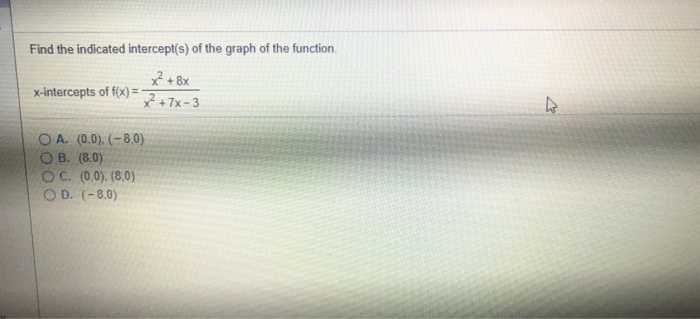Find the indicated intercept(s) of the graph of the function +8x 2 +7x-3 x-intercepts of f(x) O A. (0,0),(-8,0) O B. (8.0) O C. (0.0). (8.0) O D. (-8.0)